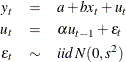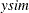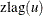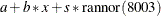# The MODEL Procedure

### Example 19.16 Simulated Method of Moments—AR(1) Process

This example illustrates how to use SMM to estimate an AR(1) regression model for the following process:In the following SAS statements,is simulated by using this model, and the endogenous variable y is set to be equal to. The MOMENT statement creates two more moments for the estimation. One is the second moment, and the other is the first-order autocovariance. The NPREOBS=10 option instructs PROC MODEL to run the simulation 10 times beforeis compared to the first observation of y. Because the initialis zero, the firstis. Without the NPREOBS option, thisis matched with the first observation of y. With NPREOBS, thisand the next nineare thrown away, and the moment match starts with the eleventhwith the first observation of y. This way, the initial values do not exert a large influence on the simulated endogenous variables.

%let nobs=500;
data ardata;
lu =0;
do i=-10 to &nobs;
x = rannor( 1011 );
e = rannor( 1011 );
u = .6 * lu + 1.5 * e;
Y = 2 + 1.5 * x + u;
lu = u;
if i > 0 then output;
end;
run;

title1 'Simulated Method of Moments for AR(1) Process';

proc model data=ardata ;
parms a b s 1 alpha .5;
instrument x;

u = alpha * zlag(u) + s * rannor( 8003 );
ysim = a + b * x + u;
y = ysim;
moment y = (2) lag1(1);

fit y / gmm npreobs=10 ndraw=10;
bound s > 0, 1 > alpha > 0;
run;


The output of the MODEL procedure is shown in Output 19.16.1:

Output 19.16.1: PROC MODEL Output

 Simulated Method of Moments for AR(1) Process

The MODEL Procedure

Model Summary
Model Variables 1
Parameters 4
Equations 3
Number of Statements 8
Program Lag Length 1

Model Variables Y a b s(1) alpha(0.5) _moment_2 _moment_1 Y

The 3 Equations to Estimate
_moment_2 = F(a, b, s, alpha)
_moment_1 = F(a, b, s, alpha)
Y = F(a(1), b(x), s, alpha)
Instruments 1 x

Nonlinear GMM Parameter Estimates
Parameter Estimate Approx Std Err t Value Approx
Pr > |t|
a 1.632798 0.1038 15.73 <.0001
b 1.513197 0.0698 21.67 <.0001
s 1.427888 0.0984 14.52 <.0001
alpha 0.543985 0.0809 6.72 <.0001